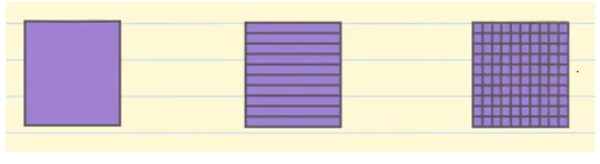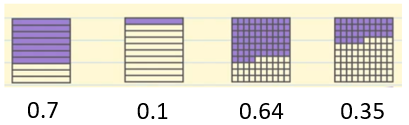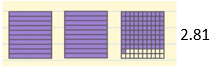Smartick is a fun way to learn math!Sep30

# What Are Decimal Numbers?

### What are decimal numbers?

Decimal numbers are used to represent numbers that are smaller than 1 unit. Decimals are written to the right of the units place separated by a period. That is the say:

Hundreds Tens Units; Tenths Hundredths Thousandths

In the image that appears below, the first square represents the unit 1. If we divide the unit into 10 equal parts (the second square), the smaller parts are tenths. If we divide the tenths into 10 equal parts or the unit 1 into 100 equal parts (the third square), the resulting parts are hundredths.Let’s look at some examples:• First example: If we divide one unit into 10 equal parts, we will have tenths. And we have colored in 7 of these parts. The way to write this is 0 units.7 tenths = 0.7.
• Second example: In the second example we also have tenths and we have colored in 1 tenth. We write this in the following form: 0 units.1 tenth = 0.1.
• Third example: In the third example we have represented hundredths, of which we have colored 6 tenths and 4 hundredths. So we write 0 units.6 tenths.4 hundredths = 0.64.
• Fourth example: In the fourth example we have represented hundredths (one unit divided by 100), of which we have colored 3 tenths and 5 hundredths. We write 0 units.3 tenths.5 hundredths = 0.35.

Let’s see another example:• Fifth example: We have 2 whole units colored and for the third unit, which is divided into hundredths, we have 8 tenths colored and 1 hundredth colored. So we write: 2 units.8 tenths 1 hundredth = 2.81

### What is the relationship between decimal numbers and fractions?

A unit is represented by 1.

Tenths are 1 unit divided into 10 equal parts = 1/10 = 0.1.

Hundredths are 1 unit divided into 100 equal parts = 1/100 = 0.01.

Thousandths are 1 unit divided into 1,000 equal parts = 1/1,000 = 0.001.

• Example of converting from a decimal to a fraction:

7.508

We focus on the last number, the 8, which occupies the thousandths place, so the denominator will have to be 1,000. And in the numerator, we write the complete number without the decimal point. So, 7.508 = 7508/1000

• Example for converting from a fraction to a decimal:

402/100

Since the denominator is 100, the last number of the numerator (2), has to be the hundredths, the previous number (0) has to be the tenths and the first number (4) has to be the units, putting the decimal point after the units. So, 402/100 = 4.02

In Smartick we offer you lots of exercises to practice decimal numbers in a fun way. We hope that you enjoy them. Register now and try it free!

Fun is our brain’s favorite way of learning
Diane Ackerman
Smartick is a fun way to learn math
• 15 fun minutes a day
• Millions of students since 2009• Mpolelo MahaoJan 12 2021, 6:36 AM

• NoxoloMar 11 2019, 1:31 PM

The app is surely going to be helpful.

• SmartickMar 12 2019, 5:34 AM